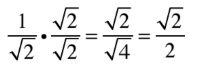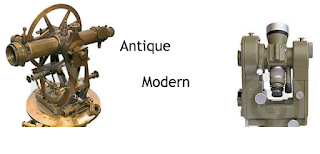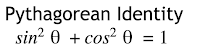# Mr. Stuckey

## Trigonometry Unit

This is the unit I have put together to teach trigonometry at Rodriguez High School. It covers standards:
• G-SRT.C.6 Understand that by similarity, side ratios in right triangles are properties of the angles in the triangle, leading to definitions of trigonometric ratios for acute angles.
• G-SRT.C.7 Explain and use the relationship between the sine and cosine of complementary angles.
• G-SRT.C.8 Use trigonometric ratios and the Pythagorean Theorem to solve right triangles in applied problems.✭
• G-SRT.C.8.1 Derive and use the trigonometric ratios for special right triangles (30°, 60°, 90°and 45°, 45°, 90°) CA
• G-SRT.D.10 (+) Prove the Laws of Sines and Cosines and use them to solve problems.
• G-SRT.D.11 (+) Understand and apply the Law of Sines and the Law of Cosines to find unknown measurements in right and non-right triangles (e.g., surveying problems, resultant forces).
• F-TF.C.8 Prove the Pythagorean identity sin²(θ) + cos²(θ) = 1 and use it to find sin(θ), cos(θ), or tan(θ) given sin(θ), cos(θ), or tan(θ) and the quadrant of the angle.
Resources that can be used throughout this unit
Trig Table
Unit Circle Trig Calculator
SOH-CAH-TOA Cheatsheet

## Activity 1 (30-45 minutes)

### Trig Investigation

Worksheets: G-SRT.C.6 Trigonometry InvestigationThe first activity is an investigation of the ratio of the sides of each triangle. Students measure the sides and angles of five different triangles and record their data in a data table. Four of the five triangles are similar and the last one is not. They are then asked to look for patterns in the data table. They should see that 4 of the 5 triangles are similar.

They then calculate the ratio of the sides in each triangle. These ratios are the sine, cosine, and tangent ratios, but they won't know that yet. They should be able to notice that the ratio of the sides of the similar triangles are the same. Then they will calculate the sin 22º using a calculator (or trig table if needed), and notice that they are the same number.

## Activity 2 (20-30 Minutes)

### Setting up Trig Ratios

Worksheets: G-SRT.C.6 SOH CAH TOATeach the mnemonic SOH CAH TOA and practice setting up the ratios. It is important to stress which angle is being used. Always ask the question "Where is theta?" first before starting anything else to promote the habit of identifying the angle being used.

## Activity 3 (30-40 minutes)

### Rationalizing the Denominator

Worksheets: Rationalizing the denominatorMy students needed to learn how to rationalize denominators because they had never done it.

## Activity 4 Solve for a Side (60-90 minutes) 2 days

### Applying Trig ratios

Worksheets: G-SRT.C.8 Solve for a side of a triangleStudents learn to solve for a side using a pre-made table to substitute for the trig functions. Calculators are not needed for this. They solve by cross multiplying. In the second page, they need to deal with square roots, whether they cancel each other out or have to rationalize them.

## Activity 5 (30-45 minutes)

### Special Right Triangles

Worksheets: Special Right Triangles and Find Missing SidesA California specific standard that has students memorize 1:2:√3 and 1:1:√2 triangles and solve for sides.

## Activity 6 (45)

### Inverse Trig Function and review

Worksheets: Find the missing Angles and Solve a Triangle
Students learn how to solve for a missing angle using inverse trig function. They then put everything together to solve a triangle for all its parts given only 3 things.

## Activity 7 (60-90 minutes) 2 days

### Applying Trig to Word Problems

Worksheets: G-SRT.C.8 Trig Word ProblemsA work problem bank with each problem given its own graphic organizer. Good for partner or group work and presentations.

## Activity 8 (30 minutes)

### Pythagorean Identities

Worksheets: F-TF.C.8 Pythagorean Trig IdentitiesA quick proof on the Pythagorean Identity and some short exercises for finding cos θ given sin θ.

## Activity (Optional) (45 Minutes)

Worksheets: G-SRT.D.11 Law of Sines and Cosines InvestigationThis is an investigation for the Law os Sines and the Law of Cosines. Students measure the sides and angles of five different triangles and record their data in a data table. Then they calculate the Law of Sines ratios and notice that they are all the same. In the next table, they calculate the Law of Cosines equations and notice that it is the same as the side opposite of the angle. Students will need practice using calculators to type the whole equation into the calculator in one line.

## Activity 9 (45 Minutes)

### Charlie's Test

Worksheets: Charlie's TestCharlie's test is a review and error correction activity in one activity. The Charlie's test is similar to the unit test, but a mediocre student has written his work on it, and the students have to correct the test for a grade.